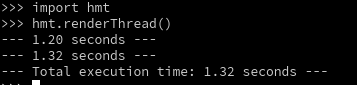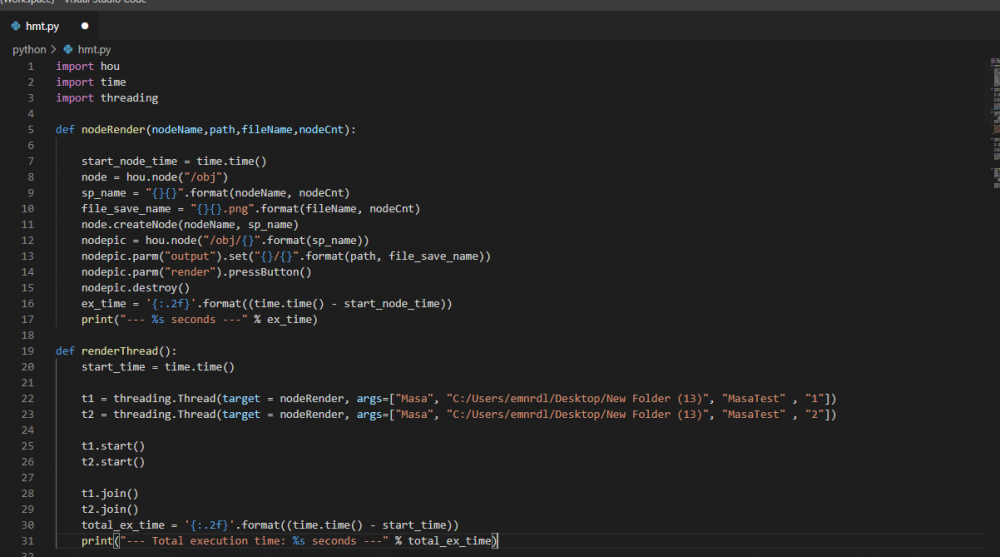# Python therading on hyton gives Qt warning

## Recommended Posts

I am trying to render 2 hda simultaneously and I decide to using python threading it works on the houduni's python shell but it don't work on hyton. What is the problem here? How can I solve this?

PythonShellhytonCode

```import hou
import time

def nodeRender(nodeName,path,fileName,nodeCnt):

start_node_time = time.time()
node = hou.node("/obj")
sp_name = "{}{}".format(nodeName, nodeCnt)
file_save_name = "{}{}.png".format(fileName, nodeCnt)
node.createNode(nodeName, sp_name)
nodepic = hou.node("/obj/{}".format(sp_name))
nodepic.parm("output").set("{}/{}".format(path, file_save_name))
nodepic.parm("render").pressButton()
nodepic.destroy()
ex_time = '{:.2f}'.format((time.time() - start_node_time))
print("--- %s seconds ---" % ex_time)

start_time = time.time()

t1 = threading.Thread(target = nodeRender, args=["Masa", "C:/Users/emnrdl/Desktop/New Folder (13)", "MasaTest" , "1"])
t2 = threading.Thread(target = nodeRender, args=["Masa", "C:/Users/emnrdl/Desktop/New Folder (13)", "MasaTest" , "2"])

t1.start()
t2.start()

t1.join()
t2.join()
total_ex_time = '{:.2f}'.format((time.time() - start_time))
print("--- Total execution time: %s seconds ---" % total_ex_time)```## Create an account

Register a new account